Скачать презентацию 3 Chemical Compounds Leaving Certificate Chemistry

4fa16bf608351cbee4b8b03e72e0909b.ppt

• Количество слайдов: 20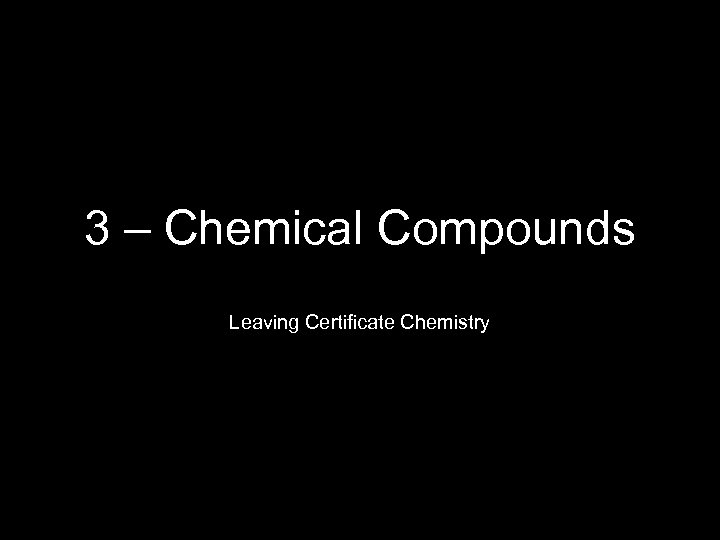3 – Chemical Compounds Leaving Certificate Chemistry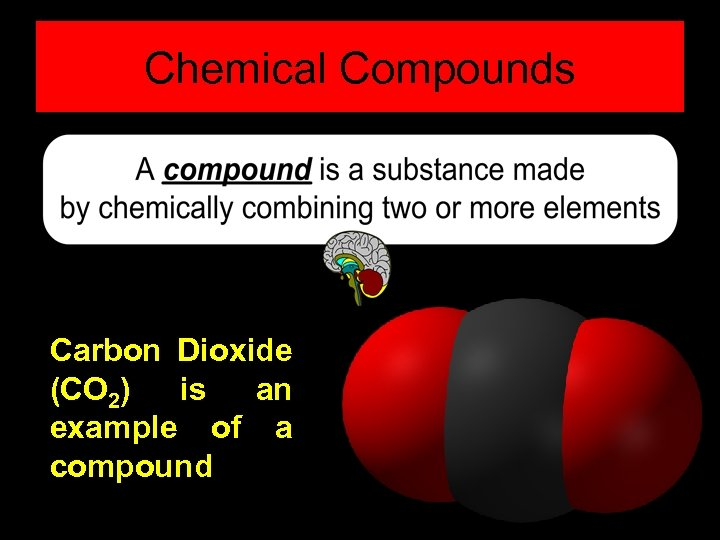Chemical Compounds Carbon Dioxide (CO 2) is an example of a compound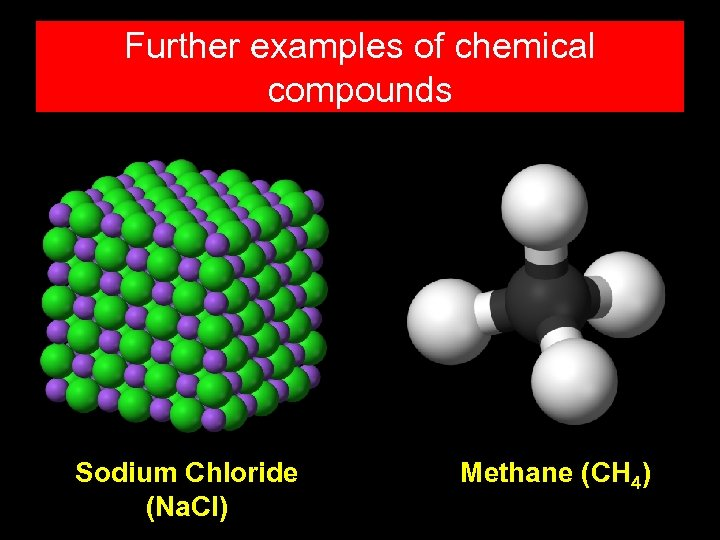Further examples of chemical compounds Sodium Chloride (Na. Cl) Methane (CH 4)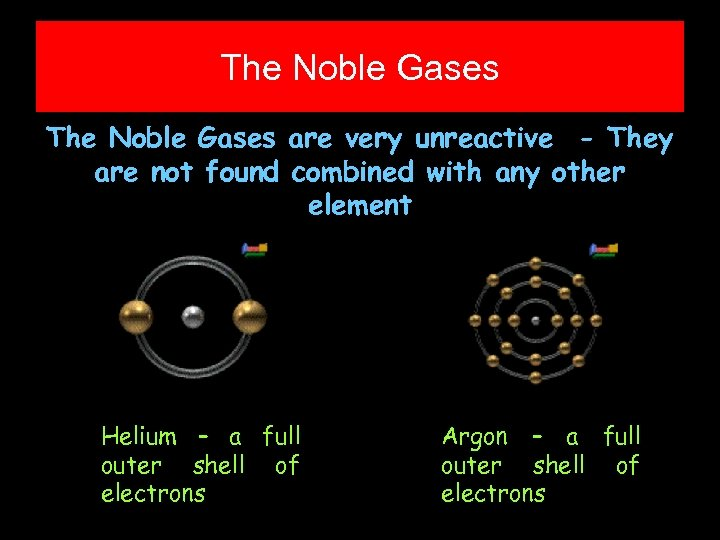The Noble Gases are very unreactive - They are not found combined with any other element Helium – a full outer shell of electrons Argon – a full outer shell of electrons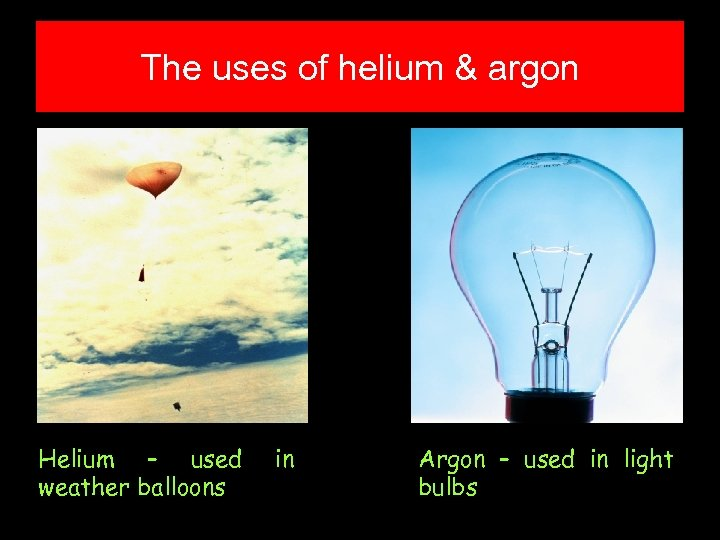The uses of helium & argon Helium – used weather balloons in Argon – used in light bulbs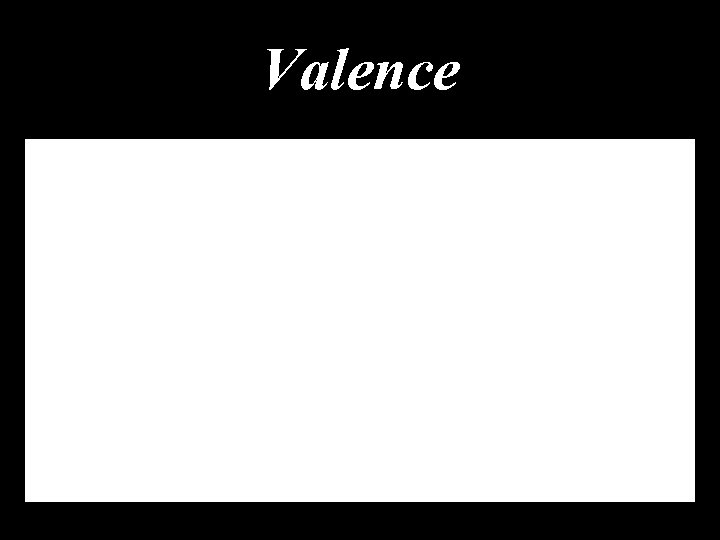Valence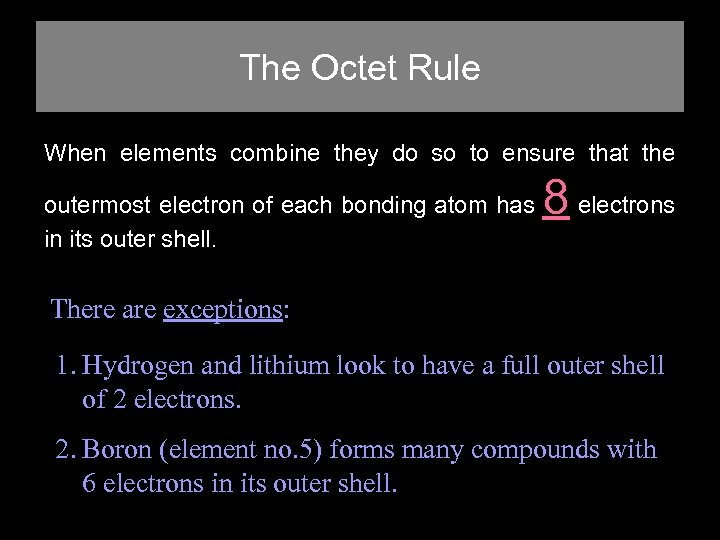The Octet Rule When elements combine they do so to ensure that the outermost electron of each bonding atom has in its outer shell. 8 electrons There are exceptions: 1. Hydrogen and lithium look to have a full outer shell of 2 electrons. 2. Boron (element no. 5) forms many compounds with 6 electrons in its outer shell.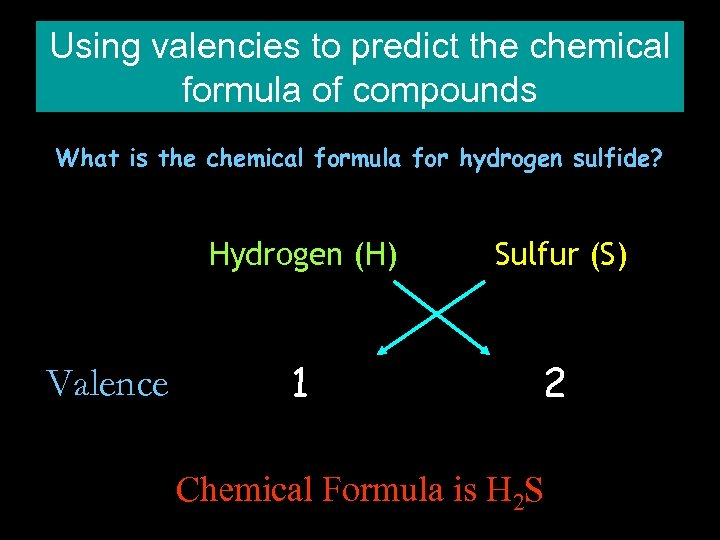Using valencies to predict the chemical formula of compounds What is the chemical formula for hydrogen sulfide? Hydrogen (H) Valence Sulfur (S) 1 2 Chemical Formula is H 2 S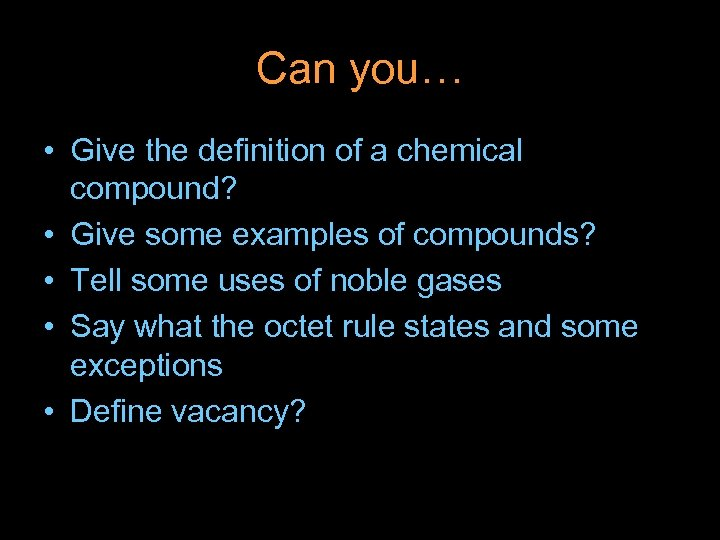Can you… • Give the definition of a chemical compound? • Give some examples of compounds? • Tell some uses of noble gases • Say what the octet rule states and some exceptions • Define vacancy?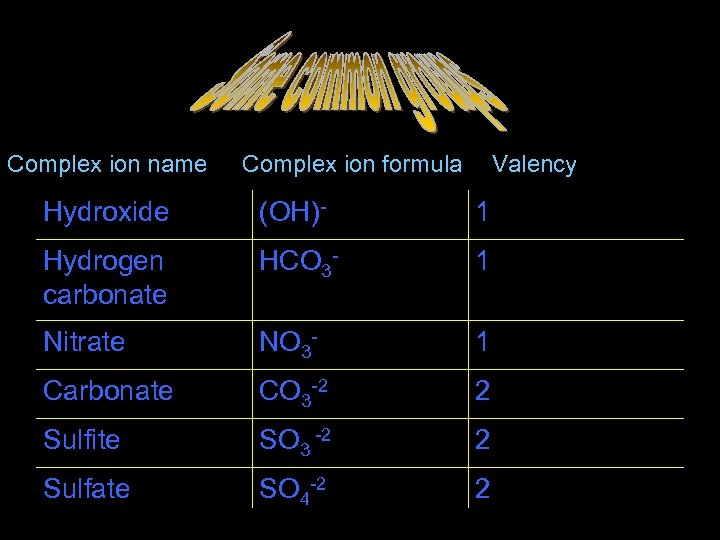Some common groups Complex ion name Complex ion formula Valency Hydroxide (OH)- 1 Hydrogen carbonate HCO 3 - 1 Nitrate NO 3 - 1 Carbonate CO 3 -2 2 Sulfite SO 3 -2 2 Sulfate SO 4 -2 2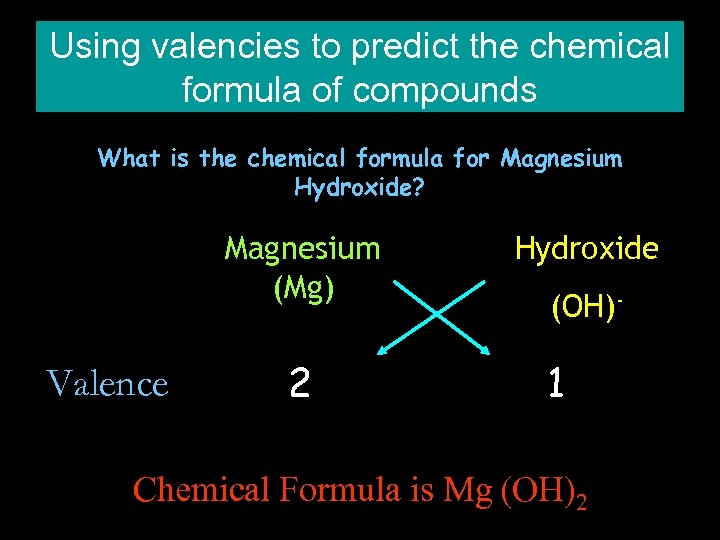Using valencies to predict the chemical formula of compounds What is the chemical formula for Magnesium Hydroxide? Magnesium (Mg) Valence 2 Hydroxide (OH)- 1 Chemical Formula is Mg (OH)2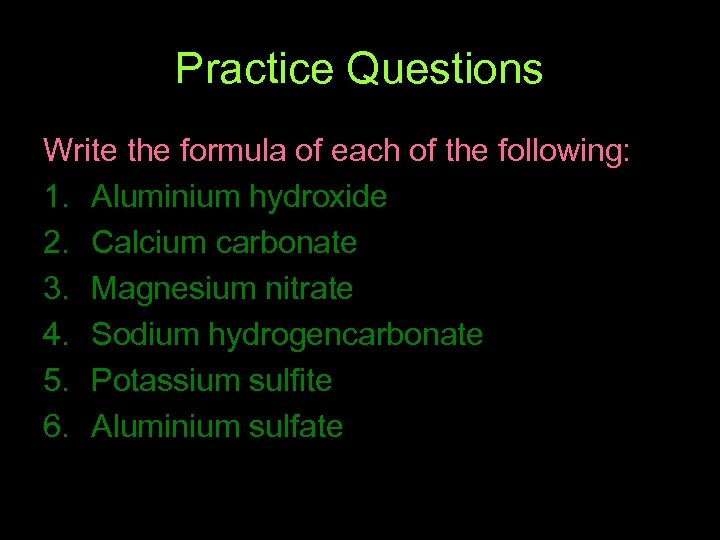Practice Questions Write the formula of each of the following: 1. Aluminium hydroxide 2. Calcium carbonate 3. Magnesium nitrate 4. Sodium hydrogencarbonate 5. Potassium sulfite 6. Aluminium sulfate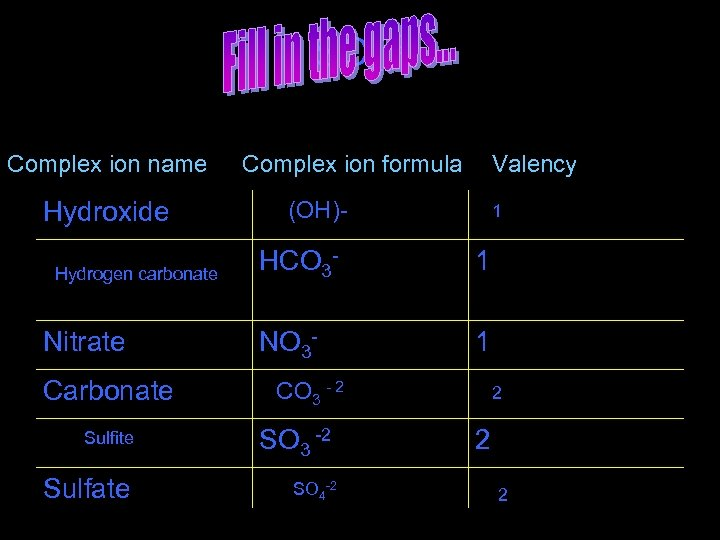SO 4 -2 Complex ion name Hydroxide Hydrogen carbonate Nitrate Carbonate Complex ion formula Valency (OH)- 1 HCO 3 - 1 NO 3 - 1 CO 3 - 2 Sulfite SO 3 -2 Sulfate SO 4 -2 2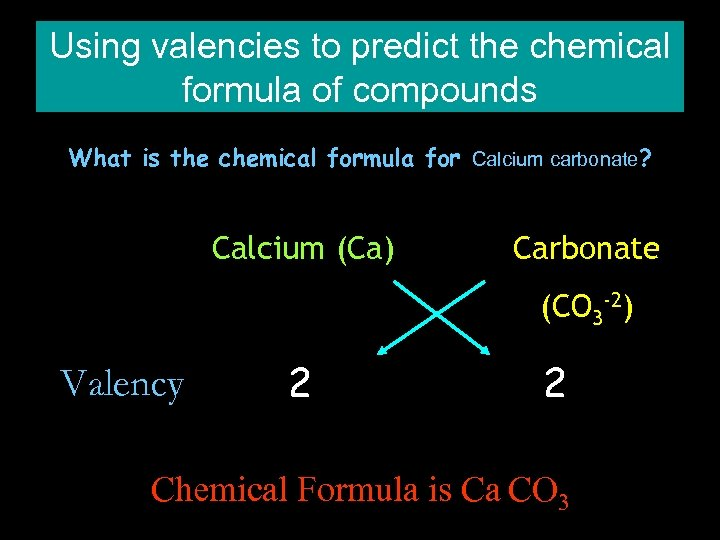Using valencies to predict the chemical formula of compounds What is the chemical formula for Calcium carbonate? Calcium (Ca) Carbonate (CO 3 -2) Valency 2 2 Chemical Formula is Ca CO 3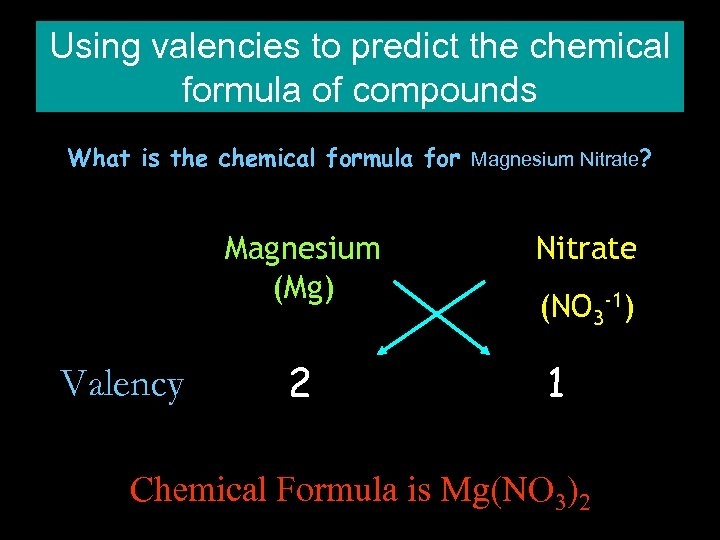Using valencies to predict the chemical formula of compounds What is the chemical formula for Magnesium Nitrate? Magnesium (Mg) Valency 2 Nitrate (NO 3 -1) 1 Chemical Formula is Mg(NO 3)2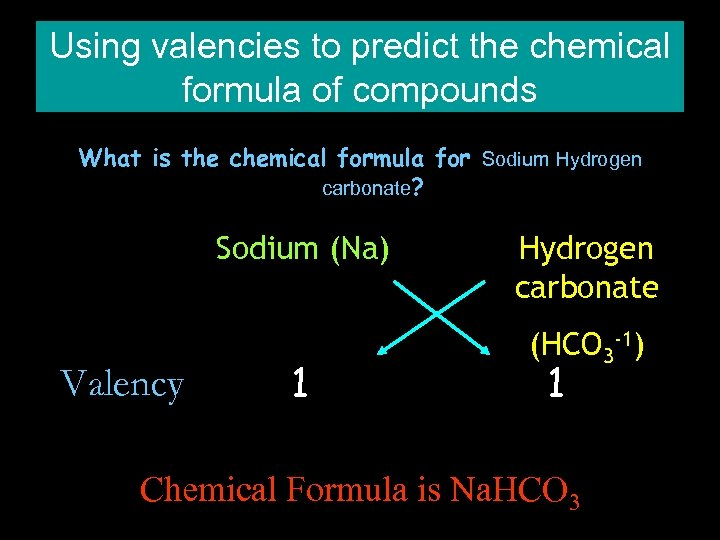Using valencies to predict the chemical formula of compounds What is the chemical formula for Sodium Hydrogen carbonate? Sodium (Na) Valency 1 Hydrogen carbonate (HCO 3 -1) 1 Chemical Formula is Na. HCO 3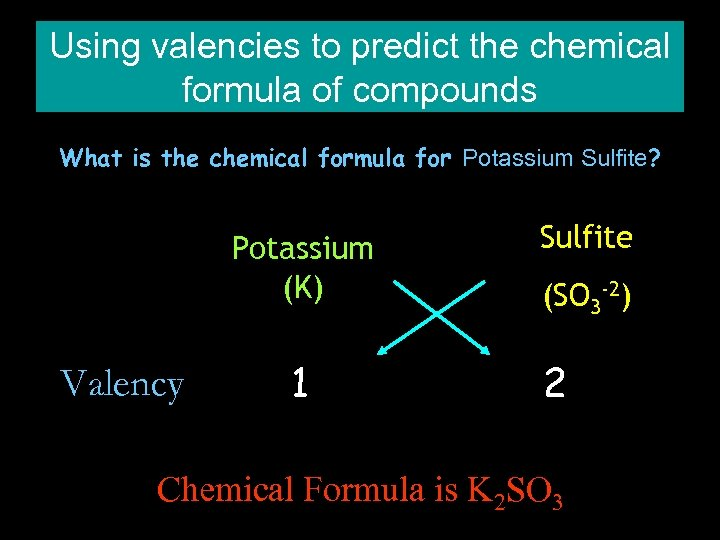Using valencies to predict the chemical formula of compounds What is the chemical formula for Potassium Sulfite? Potassium (K) Valency 1 Sulfite (SO 3 -2) 2 Chemical Formula is K 2 SO 3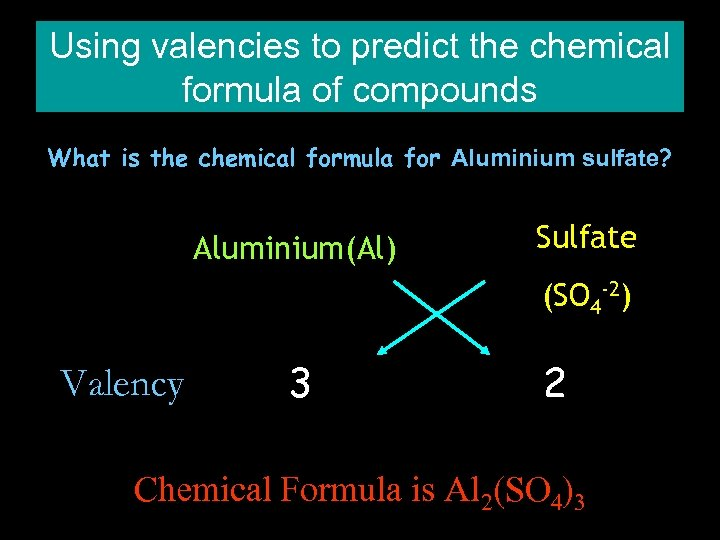Using valencies to predict the chemical formula of compounds What is the chemical formula for Aluminium sulfate? Aluminium(Al) Sulfate (SO 4 -2) Valency 3 2 Chemical Formula is Al 2(SO 4)3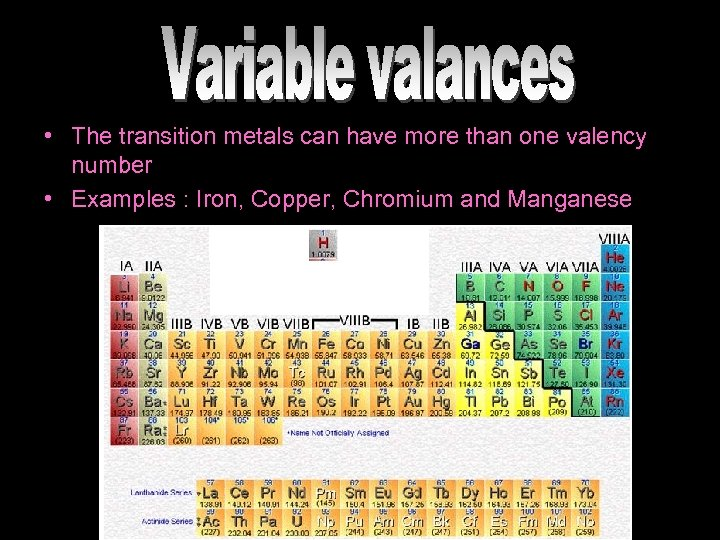• The transition metals can have more than one valency number • Examples : Iron, Copper, Chromium and Manganese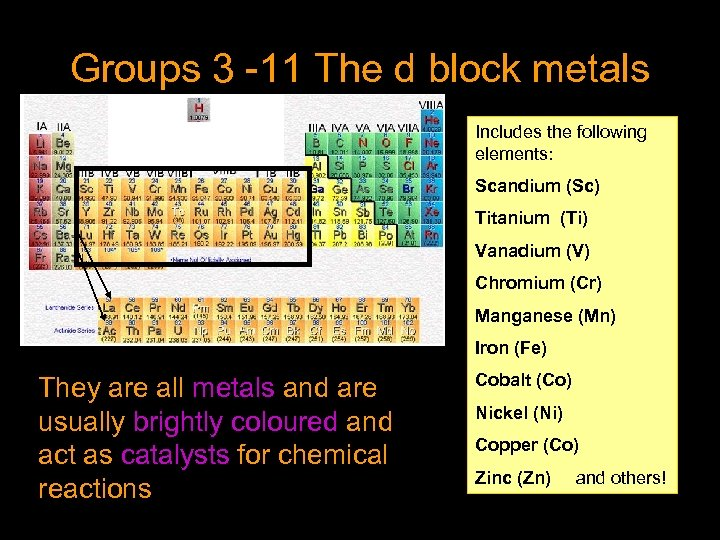Groups 3 -11 The d block metals Includes the following elements: Scandium (Sc) Titanium (Ti) Vanadium (V) Chromium (Cr) Manganese (Mn) Iron (Fe) They are all metals and are usually brightly coloured and act as catalysts for chemical reactions Cobalt (Co) Nickel (Ni) Copper (Co) Zinc (Zn) and others!